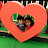Biot Savart Law of magnetic field.The magnetic field due to circular current carrying coil,Semicircular and Arc of given angle .
Lecture 1:-Gravitational Fiel,Electric field and Magnetic Field.Numerical of NEET / JEE

Lecture 2:Force on a current carrying wire in the Magnetic field intensity.Concept of drift velocity in conductor and motion of electron in conductor and the relation with the force ,magnetic field and length vector.Numerical of NEET / JEE

Lecture 3 :Torque on a rectangular coil in uniform magnetic field.The effect of torque on the coil and the unit vector in the direction of sides of rectangular coil.

Lecture 4:Motion of a charge particle at an angle with the Magnetic field intensity.The motion will be on a Helix.There is a pitch formation during the motion due to effective components of velocity along Magnetic field and perpendicular to the field.Numerical of NEET / JEE

Lecture 5: Biot-Savart Law : Magnetic Field due to Straight Wire JEE/NEET Problem.Magnetic Field due to Circular Current Carrying Loop n Arc JEE/NEET Problem also Magnetic Field on the axis of a Circular Current Loop JEE MAINS/NEET
https://youtu.be/1yUYM4Ql6B0 Senstivity
0:00:00.000,
Biot-Savrat Law

0:10:53.065,
Description of assumption defined the Law

0:18:49.886,
Magnetic field intensity at the center of circular current carrying wire

0:25:57.733,
Magnetic field intensity at the center of Semicircular Coil

0:27:20.972,
Magnetic field intensity at the center of a circular arc of angle

0:38:45.662,
Numerical of IIT JEE and NEET

source

🔥0
Click to rate this post!
[Total: 0 Average: 0]

•